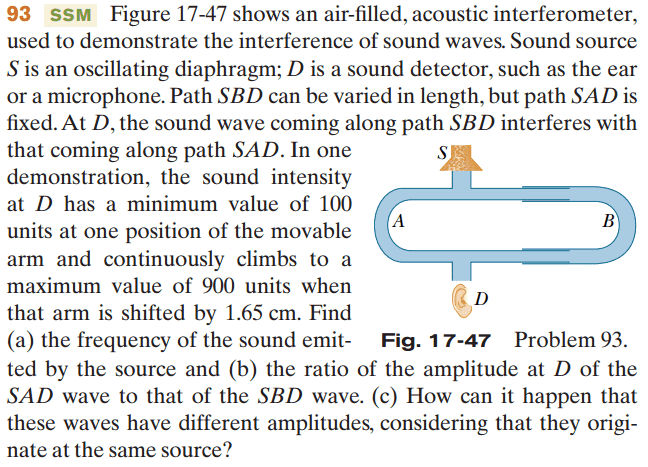# Sound waves travelling different paths

## Homework Statement## The Attempt at a Solution

So at the sound source, the waves are split in half I guess, and each of those halves travel into the different directions. Path SAD is constant and SBD is not, so the waves are changing in path SBD. Since the sound waves are changing, do I need to use derivatives somewhere?

## Answers and Replies

CWatters
Science Advisor
Homework Helper
Gold Member
No. The sound waves aren't "changing" (at least not in frequency) they just travel a longer path in SBD. Under what conditions do you get constructive and destructive interference at D? What equation relates velocity, frequency and wavelength?

No. The sound waves aren't "changing" (at least not in frequency) they just travel a longer path in SBD. Under what conditions do you get constructive and destructive interference at D? What equation relates velocity, frequency and wavelength?

When a crest of a wave meets a crest, it's constructive and when a crest meets a trought it's destructive. The wavespeed = wavelength * frequency is the equation you describe, I think.

However, I do not understand the physics that is going on. Why are you considering constructive and destructive interference? Why is the equation that relates velocity, frequency and wavelength important here? I don't physically see the relationships between the line of thinking and the problem.

CWatters
Science Advisor
Homework Helper
Gold Member
At point S the sound wave is split and half goes to the left and right. At this point (point S) both waves are in phase because they come from the same source. The path SAD and SBD are different lengths so the two waves arrive at point D at different times (eg different phase). Destructive or constructive interference will occur at point D depending on the phase difference. If the path difference is a multiple of the wave length then the two waves arrive in phase and constructive interference will occur (maximum amplitude). If the path difference is a half wavelength destructive interference will occur (minimum amplitude).

So by adjusting the path length to find where a minimum and maximium occur you can work out the length of half a wavelength and hence the wavelength. Then knowing the speed of sound in air you can calculate the frequency using V = Fλ. Just remember that moving the slider 1cm makes the path SBD 2cm longer or shorter.

As for b) and c)... the amplitude of a sound wave attenuates as it propagates through the air so if the path lengths are different they will also have different amplitude at D. This is why when destructive interference occurs the amplitude at D isn't zero but 100 units.